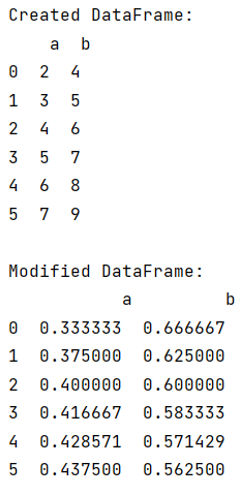# Normalize rows of pandas dataframe by their sums

Given a pandas dataframe, we have to normalize rows of it by their sums.
Submitted by Pranit Sharma, on September 20, 2022

Pandas is a special tool that allows us to perform complex manipulations of data effectively and efficiently. Inside pandas, we mostly deal with a dataset in the form of DataFrame. DataFrames are 2-dimensional data structures in pandas. DataFrames consist of rows, columns, and data.

## Normalizing pandas dataframe rows by their sums

Suppose, we have a DataFrame central and metadata. The columns are labeled with multiindex. What we have to do is to normalize each row of a particular column by the sum of that row so adding up the values in the row gives a specific value.

Rows in pandas are the different cell (column) values that are aligned horizontally and also provide uniformity. Each row can have the same or different value. Rows are generally marked with the index number but in pandas, we can also assign index names according to the needs. In pandas, we can create, read, update, and delete a column or row value.

Let us understand with the help of an example,

## Python code to normalize rows of pandas dataframe by their sums

```# Importing pandas package
import pandas as pd

# Creating a dictionary
d = {
'a': [2, 3,4,5,6,7],
'b': [4, 5,6,7,8,9]
}

# Creating a DataFrame
df = pd.DataFrame(d)

# Display Original DataFrames
print("Created DataFrame:\n",df,"\n")

# Normalizing row
df = df.div(df.sum(axis=1), axis=0)

# Display modified DataFrame
print("Modified DataFrame:\n",df)
```

Output:What's New (MCQs)

Top Interview Coding Problems/Challenges!

Languages: » C » C++ » C++ STL » Java » Data Structure » C#.Net » Android » Kotlin » SQL
Web Technologies: » PHP » Python » JavaScript » CSS » Ajax » Node.js » Web programming/HTML
Solved programs: » C » C++ » DS » Java » C#
Aptitude que. & ans.: » C » C++ » Java » DBMS
Interview que. & ans.: » C » Embedded C » Java » SEO » HR
CS Subjects: » CS Basics » O.S. » Networks » DBMS » Embedded Systems » Cloud Computing
» Machine learning » CS Organizations » Linux » DOS
More: » Articles » Puzzles » News/Updates

© https://www.includehelp.com some rights reserved.